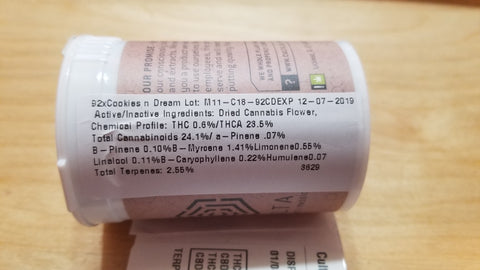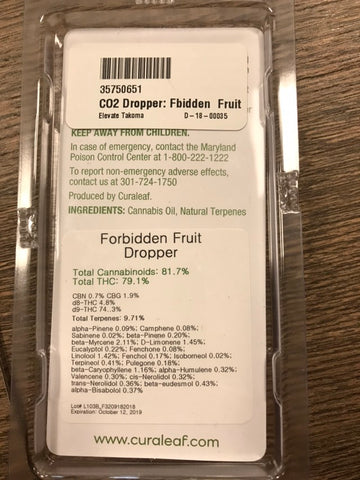# Edibles Dosing Math

If your cannabis label list only THCA you must convert it to THC before you begin the dosing calculations.

For example:

1. 1 gram is 1000mg by weight. A strain has a 20% THCA per gram. 20% of 1000mg is 200mg.
2. If there is 200mg THCA in 1 gram of flower and you have an eighth of cannabis, the total THCA in your eighth will be 200mg X 3.5 grams of cannabis = 700mg of THCA
3. To convert THCA to THC multiply your total THCA by 0.88. 700mg X 0.88 = 616mg total THC available to be extracted.
4. On average 60% of cannabinoids are extracted when making an infused oil or butter. To estimate how much THC would be extracted from your eighth multiply your total THC mg by 0.60. 616mg total THC X 0.60 = 369.6mg.
5. If you wanted each brownie to contain 10mg of THC per piece divide the total mg of THC by the number of mg that is desired per piece 369.6mg THC / 10 = 36.96 brownies. Round it up to 37 brownies.

## Flower Example

Culta 92 Cookies & Dream1 gram (g) = 1,000 milligrams (mg)
1/8th of flower is 3.5 g = 3,500 mg
23.5% THCA1. 3.5 g is 3,500 mg by weight. Culta 92 Cookies & Dream has 23.5% THCA
2. Multiply THCA with mg of flower.
3. .235 THCA X 3,500 mg = 822.5 THCA mg
4. To convert THCA mg to THC mg; multiply THCA mg by .88*
5. 822.5 THCA mg X .88 = 723.8 THC mg
6. On average 60% of cannabanoids are extracted when making an infused oil/butter
7. To estimate how much THC would be extracted from your 1/8th multiply THC mg by 60%
8. 723.8 THC mg X .60 = 434.28 THC mg after extraction
9. Using 2 cups of butter/oil. 2 cups breaks down to 32 TBSP
10. To find the mg per TBSP divide THC mg by 32 TBSP.
11. 434.28 THC mg / 32 TBSP = 13.57 THC mg per TBSP

*(Rounded) to .88, the change in molecular weight during the decarb process from THCA to THC
https://www.conflabs.com/why-0-877/

## Concentrate Example

Curaleaf Forbidden Fruit Dropper
½ g = 500 mg
Total THC: 79.1%1. ½ g is 500 mg by weight. Curaleaf Forbidden Fruit Dropper has 79.1% THC
2. Multiply 79.1% THC with 500 mg.
3. .791 THC X 500 mg = 395.5 THC mg
4. To find the mg per gummy divide THC mg with # of gummies
5. 395.5 THC mg / 32 = 12.35 THC mg per gummy

There are many online dosage calculators. I like to use the calculator on this website. Try it and see if it works for you or find another if you can’t math when you make your medicine!